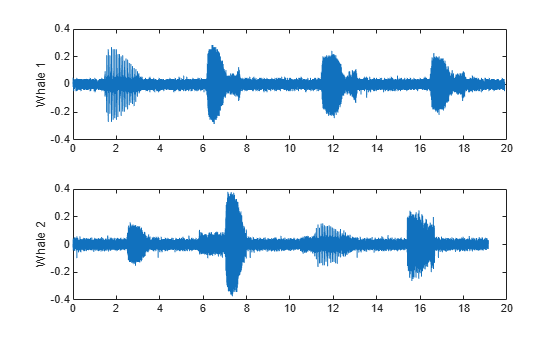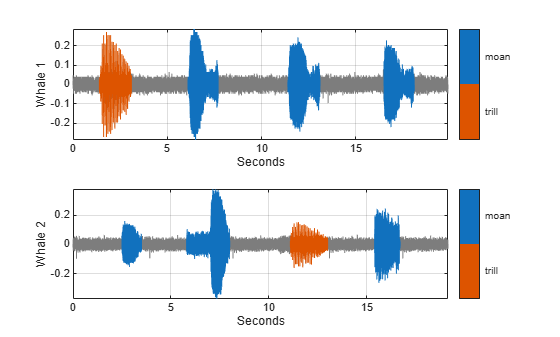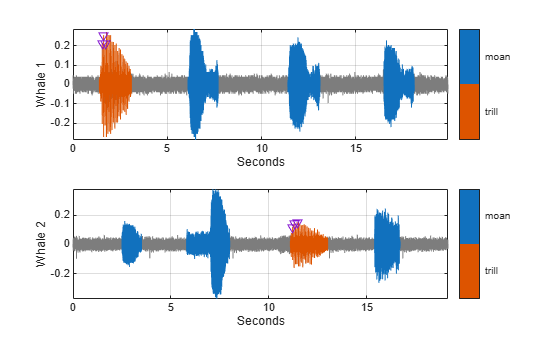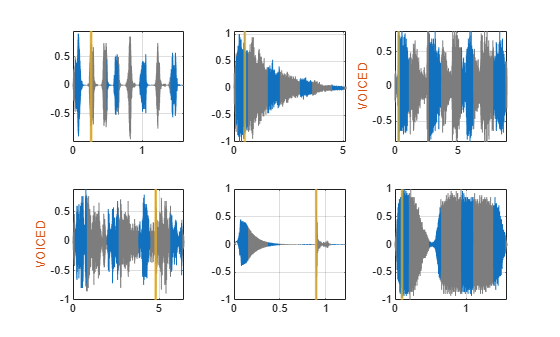# signalLabelDefinition

Create signal label definition

Since R2018b

## Description

Use `signalLabelDefinition` to create signal label definitions for data sets. The labels can correspond to attributes, regions, or points of interest. Use a vector of `signalLabelDefinition` objects to create a `labeledSignalSet`.

## Creation

### Syntax

``sld = signalLabelDefinition(name)``
``sld = signalLabelDefinition(name,Name=Value)``

### Description

````sld = signalLabelDefinition(name)` creates a signal label definition object, `sld`, with the Name property set to `name` and other properties set to default values.```

example

````sld = signalLabelDefinition(name,Name=Value)` sets Properties using name-value arguments. You can specify multiple name-value arguments. Enclose each property name in quotes.```

### Input Arguments

expand all

Label name, specified as a character vector or string scalar.

Data Types: `char` | `string`

## Properties

expand all

Name of label, specified as a character vector or string scalar.

Data Types: `char` | `string`

Type of label, specified as one of these:

• `"attribute"` — Define signal characteristics.

• `"roi"` — Define signal characteristics over regions of interest.

• `"point"` — Define signal characteristics over points of interest.

• `"attributeFeature"` — Define signal characteristics that correspond to features.

• `"roiFeature"` — Define signal characteristics over regions of interest that correspond to features.

Data Types: `char` | `string`

Data type of label, specified as `"logical"`, `"categorical"`, `"numeric"`, `"string"`, `"table"`, or `"timetable"`. When you set this property to `"categorical"`, use the Categories property to specify the array of categories. The object does not support timetable and table data types for `attributeFeature` and `roiFeature` labels.

Data Types: `char` | `string`

Label category names, specified as a string array or a cell array of character vectors. The array must have unique elements. This property applies only when the LabelDataType property is set to `"categorical"`.

Example: `LabelDataType="categorical",Categories=["apple","orange"]`

Data Types: `char` | `string`

Data type of ROI limits, specified as either `"double"` or `"duration"`. This property applies only when LabelType is set to `"roi"`.

Data Types: `char` | `string`

Data type of point locations, specified as either `"double"` or `"duration"`. This property applies only when LabelType is set to `"point"`.

Data Types: `char` | `string`

Validation function, specified as a function handle and used when setting label values in a `labeledSignalSet` object. This property applies only when LabelDataType is set to `"categorical"`, `"logical"`, `"numeric"`, `"table"`, or `"timetable"`. If not specified, the function checks only that its input values are of the correct data type. If LabelDataType is set to `"categorical"`, the function checks that the input is one of the values specified using Categories. The function takes an input value and returns `true` if the value is valid and `false` if the value is invalid.

Example: `LabelDataType="numeric",DefaultValue=1,ValidationFunction=@(x)x<2`

Data Types: `function_handle`

Default value of label, specified as a value of the type specified using LabelDataType. If LabelDataType is set to `"categorical"`, then DefaultValue must be one of the values specified using Categories.

Example: `LabelDataType="categorical",Categories=["apple","orange"],DefaultValue="apple"`

Data Types: `char` | `double` | `logical` | `string` | `table`

Label description, specified as a character vector or string scalar.

Example: `Description="Patient is asleep"`

Data Types: `char` | `string`

Label tag identifier, specified as a character vector or string scalar. Use this property to identify the same label in a larger labeling scheme or public labeling set.

Example: `Tag="Peak1"`

Data Types: `char` | `string`

Array of sublabels, specified as a signal label definition object. To specify more than one sublabel, set this property to a vector of signal label definition objects. Use this property to create a relationship between a parent label and its children. If LabelType is set to `"attributeFeature"` or `"roiFeature"`, then this property does not apply.

Note

Sublabels cannot have sublabels.

Example: `Sublabels=[signalLabelDefinition("negative"),signalLabelDefinition("positive")]`

Frame size, specified as a numeric scalar. You must specify `FrameSize` when LabelType is set to `"roiFeature"`.

Example: `FrameSize=50`

Data Types: `double`

Overlap length of adjacent frames, specified as a numeric scalar. To enable this property, set LabelType to `"roiFeature"`. You cannot specify `FrameOverlapLength` and `FrameRate` simultaneously. If you do not specify `FramerOverlapLength`, then the object assumes the overlap length to be zero.

Example: `FrameSize=50,FrameOverlapLength=5`

Data Types: `double`

Frame rate, specified as a numeric scalar. To enable this property, set LabelType to `"roiFeature"`. You cannot specify `FrameRate` and `FrameOverlapLength` simultaneously. If you do not specify `FrameRate`, then the object assumes no overlap between frames.

Example: `FrameSize=50,FrameRate=45`

Data Types: `double`

## Object Functions

 `labelDefinitionsHierarchy` Get hierarchical list of label and sublabel names `labelDefinitionsSummary` Get summary table of signal label definitions

## Examples

collapse all

Consider a set of whale sound recordings. The recorded whale sounds consist of trills and moans. Trills sound like series of clicks. Moans are low-frequency cries similar to the sound made by a ship's horn. You want to look at each signal and label it to identify the whale type, the trill regions, and the moan regions. For each trill region, you also want to label the signal peaks higher than a certain threshold.

Signal Label Definitions

Define an attribute label to store whale types. The possible categories are blue whale, humpback whale, and white whale.

```dWhaleType = signalLabelDefinition('WhaleType',... 'LabelType','attribute',... 'LabelDataType','categorical',... 'Categories',{'blue','humpback','white'},... 'Description','Whale type'); ```

Define a region-of-interest (ROI) label to capture moan regions. Define another ROI label to capture trill regions.

```dMoans = signalLabelDefinition('MoanRegions',... 'LabelType','roi',... 'LabelDataType','logical',... 'Description','Regions where moans occur'); dTrills = signalLabelDefinition('TrillRegions',... 'LabelType','roi',... 'LabelDataType','logical',... 'Description','Regions where trills occur'); ```

Finally, define a point label to capture the trill peaks. Set this label as a sublabel of the `dTrills` definition.

```dTrillPeaks = signalLabelDefinition('TrillPeaks',... 'LabelType','point',... 'LabelDataType','numeric',... 'Description','Trill peaks'); dTrills.Sublabels = dTrillPeaks;```

Labeled Signal Set

Create a `labeledSignalSet` with the whale signals and the label definitions. Add label values to identify the whale type, the moan and trill regions, and the peaks of the trills.

```load labelwhalesignals lbldefs = [dWhaleType dMoans dTrills]; lss = labeledSignalSet({whale1 whale2},lbldefs,'MemberNames',{'Whale1','Whale2'}, ... 'SampleRate',Fs,'Description','Characterize whale song regions'); ```

Visualize the label hierarchy and label properties using `labelDefinitionsHierarchy` and `labelDefinitionsSummary`.

`labelDefinitionsHierarchy(lss)`
```ans = 'WhaleType Sublabels: [] MoanRegions Sublabels: [] TrillRegions Sublabels: TrillPeaks ' ```
`labelDefinitionsSummary(lss)`
```ans=3×9 table LabelName LabelType LabelDataType Categories ValidationFunction DefaultValue Sublabels Tag Description ______________ ___________ _____________ ____________ __________________ ____________ ___________________________ ___ ____________________________ "WhaleType" "attribute" "categorical" {3x1 string} {["N/A" ]} {0x0 double} {0x0 double } "" "Whale type" "MoanRegions" "roi" "logical" {["N/A" ]} {0x0 double} {0x0 double} {0x0 double } "" "Regions where moans occur" "TrillRegions" "roi" "logical" {["N/A" ]} {0x0 double} {0x0 double} {1x1 signalLabelDefinition} "" "Regions where trills occur" ```

The signals in the loaded data correspond to songs of two blue whales. Set the `'WhaleType'` values for both signals.

```setLabelValue(lss,1,'WhaleType','blue'); setLabelValue(lss,2,'WhaleType','blue');```

Visualize the `'Labels'` property. The table has the newly added `'WhaleType'` values for both signals.

`lss.Labels `
```ans=2×3 table WhaleType MoanRegions TrillRegions _________ ___________ ____________ Whale1 blue {0x2 table} {0x3 table} Whale2 blue {0x2 table} {0x3 table} ```

Visualize Region Labels

Visualize the whale songs to identify the trill and moan regions.

```subplot(2,1,1) plot((0:length(whale1)-1)/Fs,whale1) ylabel('Whale 1') subplot(2,1,2) plot((0:length(whale2)-1)/Fs,whale2) ylabel('Whale 2')```Moan regions are sustained low-frequency wails.

• `whale1` has moans centered at about 7 seconds, 12 seconds, and 17 seconds.

• `whale2` has moans centered at about 3 seconds, 7 seconds, and 16 seconds.

Add the moan regions to the labeled set. Specify the ROI limits in seconds and the label values.

```moanRegionsWhale1 = [6.1 7.7; 11.4 13.1; 16.5 18.1]; mrsz1 = [size(moanRegionsWhale1,1) 1]; setLabelValue(lss,1,'MoanRegions',moanRegionsWhale1,true(mrsz1)); moanRegionsWhale2 = [2.5 3.5; 5.8 8; 15.4 16.7]; mrsz2 = [size(moanRegionsWhale2,1) 1]; setLabelValue(lss,2,'MoanRegions',moanRegionsWhale2,true(mrsz2));```

Trill regions have distinct bursts of sound punctuated by silence.

• `whale1` has a trill centered at about 2 seconds.

• `whale2` has a trill centered at about 12 seconds.

Add the trill regions to the labeled set.

```trillRegionWhale1 = [1.4 3.1]; trsz1 = [size(trillRegionWhale1,1) 1]; setLabelValue(lss,1,'TrillRegions',trillRegionWhale1,true(trsz1)); trillRegionWhale2 = [11.1 13]; trsz2 = [size(trillRegionWhale1,1) 1]; setLabelValue(lss,2,'TrillRegions',trillRegionWhale2,true(trsz2));```

Create a `signalMask` object for each whale song and use it to visualize and label the different regions. For better visualization, change the label values from logical to categorical.

```mr1 = getLabelValues(lss,1,'MoanRegions'); mr1.Value = categorical(repmat("moan",mrsz1)); tr1 = getLabelValues(lss,1,'TrillRegions'); tr1.Value = categorical(repmat("trill",trsz1)); msk1 = signalMask([mr1;tr1],'SampleRate',Fs); subplot(2,1,1) plotsigroi(msk1,whale1) ylabel('Whale 1') hold on mr2 = getLabelValues(lss,2,'MoanRegions'); mr2.Value = categorical(repmat("moan",mrsz2)); tr2 = getLabelValues(lss,2,'TrillRegions'); tr2.Value = categorical(repmat("trill",trsz2)); msk2 = signalMask([mr2;tr2],'SampleRate',Fs); subplot(2,1,2) plotsigroi(msk2,whale2) ylabel('Whale 2') hold on```Visualize Point Labels

Label three peaks for each trill region. For point labels, you specify the point locations and the label values. In this example, the point locations are in seconds.

```peakLocsWhale1 = [1.553 1.626 1.7]; peakValsWhale1 = [0.211 0.254 0.211]; setLabelValue(lss,1,{'TrillRegions','TrillPeaks'}, ... peakLocsWhale1,peakValsWhale1,'LabelRowIndex',1); subplot(2,1,1) plot(peakLocsWhale1,peakValsWhale1,'v') hold off peakLocsWhale2 = [11.214 11.288 11.437]; peakValsWhale2 = [0.119 0.14 0.15]; setLabelValue(lss,2,{'TrillRegions','TrillPeaks'}, ... peakLocsWhale2,peakValsWhale2,'LabelRowIndex',1); subplot(2,1,2) plot(peakLocsWhale2,peakValsWhale2,'v') hold off```Explore Label Values

Explore the label values using `getLabelValues`.

`getLabelValues(lss)`
```ans=2×3 table WhaleType MoanRegions TrillRegions _________ ___________ ____________ Whale1 blue {3x2 table} {1x3 table} Whale2 blue {3x2 table} {1x3 table} ```

Retrieve the moan regions for the first member of the labeled set.

`getLabelValues(lss,1,'MoanRegions')`
```ans=3×2 table ROILimits Value ____________ _____ 6.1 7.7 {} 11.4 13.1 {} 16.5 18.1 {} ```

Use a second output argument to list the sublabels of a label.

`[value,valueWithSublabel] = getLabelValues(lss,1,'TrillRegions')`
```value=1×2 table ROILimits Value __________ _____ 1.4 3.1 {} ```
```valueWithSublabel=1×3 table ROILimits Value Sublabels TrillPeaks __________ _____ ___________ 1.4 3.1 {} {3x2 table} ```

To retrieve the values in a sublabel, express the label name as a two-element array.

`getLabelValues(lss,1,{'TrillRegions','TrillPeaks'})`
```ans=3×2 table Location Value ________ __________ 1.553 {[0.2110]} 1.626 {[0.2540]} 1.7 {[0.2110]} ```

Find the value of the third trill peak corresponding to the second member of the set.

```getLabelValues(lss,2,{'TrillRegions','TrillPeaks'}, ... 'LabelRowIndex',1,'SublabelRowIndex',3)```
```ans=1×2 table Location Value ________ __________ 11.437 {[0.1500]} ```

Specify the path to a set of audio signals included as MAT-files with MATLAB®. Each file contains a signal variable and a sample rate. List the names of the files.

```folder = fullfile(matlabroot,"toolbox","matlab","audiovideo"); lst = dir(append(folder,"/*.mat")); nms = {lst(:).name}'```
```nms = 7x1 cell {'chirp.mat' } {'gong.mat' } {'handel.mat' } {'laughter.mat'} {'mtlb.mat' } {'splat.mat' } {'train.mat' } ```

Create a signal datastore that points to the specified folder. Set the sample rate variable name to `Fs`, which is common to all files. Generate a subset of the datastore that excludes the file `mtlb.mat`. Use the subset datastore as the source for a `labeledSignalSet` object.

```sds = signalDatastore(folder,"SampleRateVariableName","Fs"); sds = subset(sds,~strcmp(nms,"mtlb.mat")); lss = labeledSignalSet(sds);```

Create three label definitions to label the signals:

• Define a logical attribute label that is true for signals that contain human voices.

• Define a numeric point label that marks the location and amplitude of the maximum of each signal.

• Define a categorical region-of-interest (ROI) label to pick out nonoverlapping, uniform-length random regions of each signal.

Add the signal label definitions to the labeled signal set.

```vc = signalLabelDefinition("Voice",'LabelType','attribute', ... 'LabelDataType','logical','DefaultValue',false); mx = signalLabelDefinition("Maximum",'LabelType','point', ... 'LabelDataType','numeric'); rs = signalLabelDefinition("RanROI",'LabelType','ROI', ... 'LabelDataType','categorical','Categories',["ROI" "other"]); addLabelDefinitions(lss,[vc mx rs])```

Label the signals:

• Label `'handel.mat'` and `'laughter.mat'` as having human voices.

• Use the `islocalmax` function to find the maximum of each signal. Label its location and value.

• Use the `randROI` function to generate as many regions of length N/10 samples as can fit in a signal of length N given a minimum separation of N/6 samples between regions. Label their locations and assign them to the `ROI` category.

When labeling points and regions, convert sample values to time values. Subtract 1 to account for MATLAB® array indexing and divide by the sample rate.

```kj = 1; while hasdata(sds) [sig,info] = read(sds); fs = info.SampleRate; [~,fn] = fileparts(info.FileName); if fn=="handel" || fn=="laughter" setLabelValue(lss,kj,"Voice",true) end xm = find(islocalmax(sig,'MaxNumExtrema',1)); setLabelValue(lss,kj,"Maximum",(xm-1)/fs,sig(xm)) N = length(sig); rois = randROI(N,round(N/10),round(N/6)); setLabelValue(lss,kj,"RanROI",(rois-1)/fs,repelem("ROI",size(rois,1))) kj = kj+1; end```

Verify that only two signals contain voices.

`countLabelValues(lss,"Voice")`
```ans=2×3 table Voice Count Percent _____ _____ _______ false 4 66.667 true 2 33.333 ```

Verify that two signals have a maximum amplitude of 1.

`countLabelValues(lss,"Maximum")`
```ans=5×4 table Maximum Count Percent MemberCount ______________________ _____ _______ ___________ 0.80000000000000004441 1 16.667 1 0.89113331915798421612 1 16.667 1 0.94730769230769229505 1 16.667 1 1 2 33.333 2 1.0575668990330560071 1 16.667 1 ```

Verify that each signal has four nonoverlapping random regions of interest.

`countLabelValues(lss,"RanROI")`
```ans=2×4 table RanROI Count Percent MemberCount ______ _____ _______ ___________ ROI 24 100 6 other 0 0 0 ```

Create two datastores with the data in the labeled signal set:

`[sd,ld] = createDatastores(lss,["Voice" "RanROI" "Maximum"]);`

Use the information in the datastores to plot the signals and display their labels.

• Use a `signalMask` object to highlight the regions of interest in blue.

• Plot yellow lines to mark the locations of the maxima.

• Add a red axis label to the signals that contain human voices.

```tiledlayout flow while hasdata(sd) [sg,nf] = read(sd); lbls = read(ld); nexttile msk = signalMask(lbls{:}.RanROI{:},'SampleRate',nf.SampleRate); plotsigroi(msk,sg) colorbar off xlabel('') xline(lbls{:}.Maximum{:}.Location, ... 'LineWidth',2,'Color','#EDB120') if lbls{:}.Voice{:} ylabel('VOICED','Color','#D95319') end end``````function roilims = randROI(N,wid,sep) num = floor((N+sep)/(wid+sep)); hq = histcounts(randi(num+1,1,N-num*wid-(num-1)*sep),(1:num+2)-1/2); roilims = (1 + (0:num-1)*(wid+sep) + cumsum(hq(1:num)))' + [0 wid-1]; end```

## Version History

Introduced in R2018b